﻿ 基于BP神经网络的电动汽车负荷预测 Electric Vehicle Load Forecasting Based on BP Neural Network

Journal of Electrical Engineering
Vol. 07  No. 01 ( 2019 ), Article ID: 28648 , 12 pages
10.12677/JEE.2019.71002

Electric Vehicle Load Forecasting Based on BP Neural Network

Xin Lan

The Affiliated Middle School of Southwest Jiaotong University, Chengdu SichuanReceived: Jan. 3rd, 2019; accepted: Jan. 18th, 2019; published: Jan. 25th, 2019ABSTRACT

Large-scale electric vehicles (EVs) access will cause serious impact on the power grid and affect the reliable operation. In order to reduce the damage to the balance of the power grid caused by the simultaneous access of EVs, the research on the electrical characteristics of EVs based on large data becomes the key to enhance the reliability and safety of the power grid. In this paper, through the characteristics and related parameters of EVs’ operation, the impact of different factors on charging load is analyzed, and the charging load models of various types of EVs are studied. On this basis, combined with BP neural network algorithm, the development trend of EV is forecasted, the charging load forecasting model of EV is established, and the power consumption of EV in the next few years is estimated, which provides a reference basis for grid optimization and power distribution.

Keywords:Electric Vehicle, Big Data Analysis, Charging Load Models, BP Neural Network, Load Forecasting1. 引言

2.充电负荷影响因素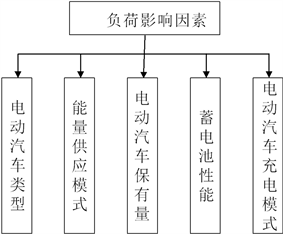Figure 1. Influencing factors of EV charging load

2.1. 电动汽车类型

2.2. 能量供应模式

2.3. 电动汽车保有量

2.4. 蓄电池特性

1) 铅酸蓄电池

2) 锂电池

2.5. 电动汽车的充电模式

3. 电动汽车充电负荷模型

3.1. 充电方式及充电时间

3.2. 电动汽车日行驶距离及初始电量

$Q=\left(1-\frac{L}{{L}_{\mathrm{max}}}\right)×100%$ (1)

3.3. 电动汽车充电功率及时长

${t}_{s}=\frac{LW}{P}$ (2)

3.4. 蒙特卡洛模拟法

3.5. 电动汽车充电负荷预测

${P}_{i}=\sum _{n=1}^{N}{p}_{n,i}$ (3)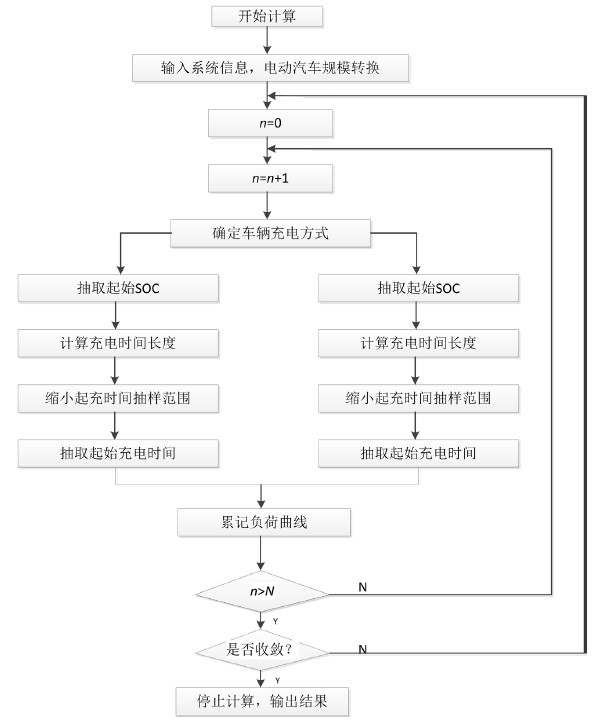Figure 2. Charging load solution flow chart of electric bus

4. 电动汽车数量预测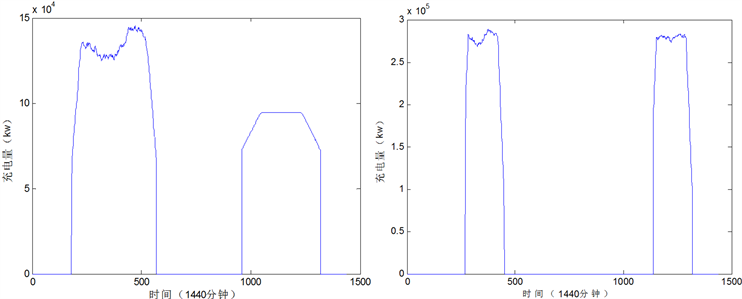(a) 公交车日充电负荷 (b) 出租车(大)日充电负荷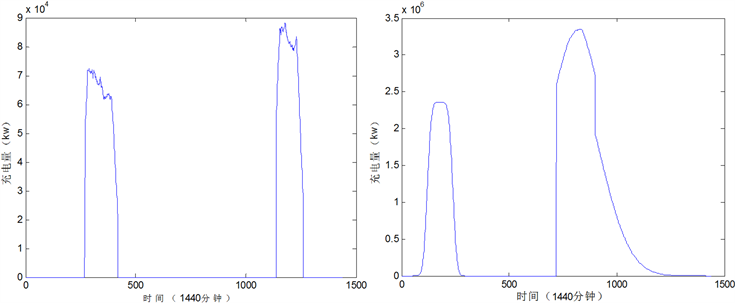(c) 出租车(小)日充电负荷 (d) 私家车日充电负荷(工作)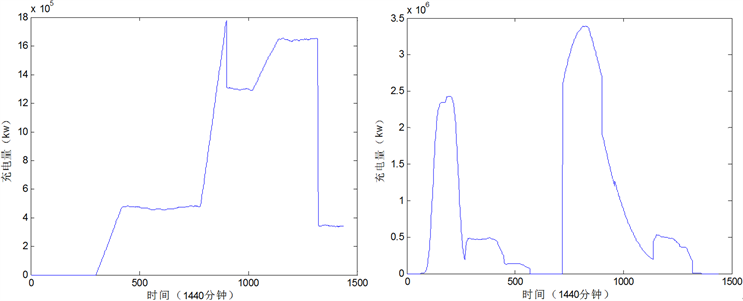(e) 私家车日充电负荷(周末) (f) 电动汽车日充电负荷(工作)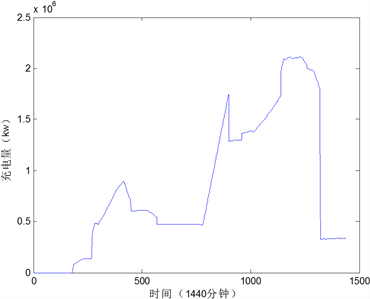(g) 电动汽车日充电负荷(周末)

Figure 3. Charging load curve of various types of EVs in Beijing 2017

Table 4. Historical data of EV quantityTable 7. EV quantity forecast from 2018 to 2020

5. 负荷预测结果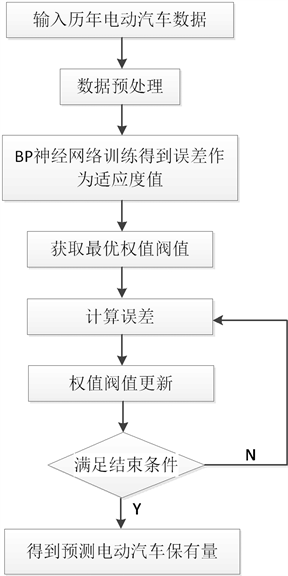Figure 4. Flow chart of EV quantity prediction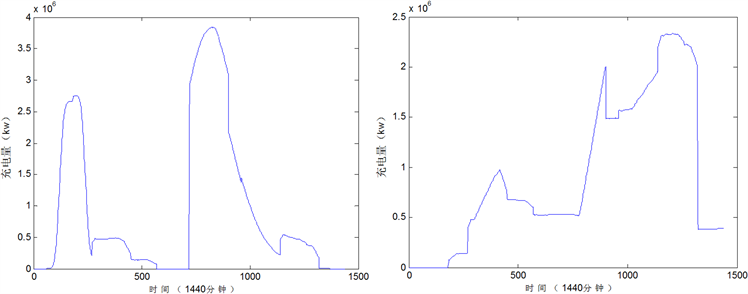(a) 2018工作日充电负荷 (b) 2018周末日充电负荷(c) 2019工作日充电负荷 (d) 2019周末日充电负荷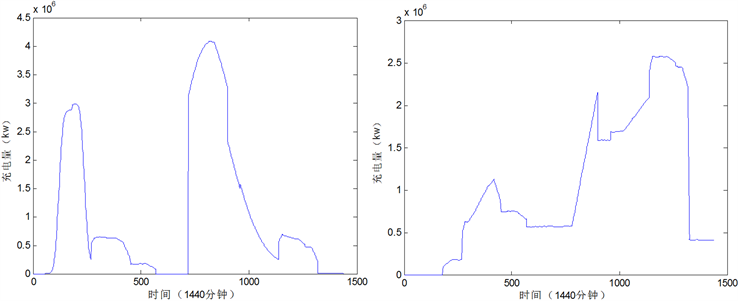(e) 2019工作日充电负荷 (f) 2019周末日充电负荷

Figure 5. Total daily load prediction curve of EVs

6. 结束语

Electric Vehicle Load Forecasting Based on BP Neural Network[J]. 电气工程, 2019, 07(01): 7-18. https://doi.org/10.12677/JEE.2019.71002

1. 1. 欧阳明高. 我国节能与新能源汽车发展战略与对策[J]. 汽车工程, 2006, 28(4): 317-321.

2. 2. 赵俊华, 文福拴, 杨爱民, 等. 电动汽车对电力系统的影响及其调度与控制问题[J]. 电力系统自动化, 2012, 35(14): 2-10.

3. 3. 许晓明. 计及电动汽车的配电网规划研究[D]: [硕士学位论文]. 北京: 华北电力大学, 2012.

4. 4. Rotering, N. and Ilic, M. (2011) Optimal Charge Control of Plug-In Hybrid Electric Vehicles in Deregulated Electricity Markets. IEEE Transactions on Power Systems, 26, 1021-1029. https://doi.org/10.1109/TPWRS.2010.2086083

5. 5. 寇凌峰. 电动汽车大规模接入对电网的影响分析[D]: [硕士学位论文]. 北京: 华北电力大学(北京), 2011.

6. 6. 冯国峥. 北京地区电动汽车发展预测与电力公司应对措施[D]: [硕士学位论文]. 北京: 华北电力大学(北京), 2011.

7. 7. 孟颖. 规模化电动汽车充电负荷特性研究及其对电网的影响[D]: [硕士学位论文]. 北京: 华北电力大学(北京), 2016.